trajectory

Also found in: Dictionary, Thesaurus, Medical, Acronyms, Wikipedia.

trajectory

1. the path described by an object moving in air or space under the influence of such forces as thrust, wind resistance, and gravity, esp the curved path of a projectile
2. Geometry a curve that cuts a family of curves or surfaces at a constant angle
Collins Discovery Encyclopedia, 1st edition © HarperCollins Publishers 2005

Trajectory

The curve described by a body moving through space, as of a meteor through the atmosphere, a planet around the Sun, a projectile fired from a gun, or a rocket in flight. In general, the trajectory of a body in a gravitational field is a conic section—ellipse, hyperbola, or parabola—depending on the energy of motion. The trajectory of a shell or rocket fired from the ground is a portion of an ellipse with the Earth's center as one focus; however, if the altitude reached is not great, the effect of gravity is essentially constant, and the parabola is a good approximation. See Ballistics

McGraw-Hill Concise Encyclopedia of Physics. © 2002 by The McGraw-Hill Companies, Inc.

trajectory

(tră-jek -tŏ-ree) The curved path followed by a moving spacecraft, rocket, etc., under the forces of gravity, lift, and/or drag.
Collins Dictionary of Astronomy © Market House Books Ltd, 2006
The following article is from The Great Soviet Encyclopedia (1979). It might be outdated or ideologically biased.

Trajectory

in exterior ballistics, the curve traced in space by the center of mass of a shell or rocket from the moment the projectile leaves the muzzle of the gun or the guide or barrel of the launcher and loses contact with the muzzle, guide, or barrel. The shape of a trajectory depends on the earth’s gravity and rotation as well as on the aerodynamic and reaction forces acting on the shell or rocket in flight.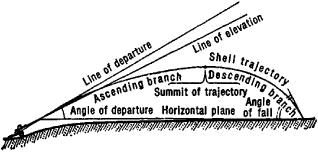Figure 1. Elements of a trajectory

Shells move along ballistic trajectories (Figure 1). In the case of small angles of fall (up to 20°), we speak of shallow trajectories and very low-angle fire; for angles of fall greater than 20°, we speak of steep trajectories and high-angle fire. When fired at airborne targets, antiaircraft shells—unlike artillery shells—have trajectories with only an ascending branch; the trajectories of rocket shells and rocket-assisted projectiles (for example, antitank rockets) have one or more powered phases, in which the rocket engines operate, and several free-flight phases. When the total length of the powered phases is small compared with the whole trajectory, the trajectory differs little from a ballistic trajectory; if the flight is controlled over the entire length of the trajectory or a substantial part of it, the trajectory differs considerably from a ballistic one.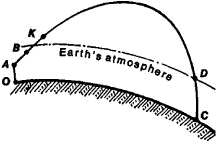Figure 2. The trajectory of a ballistic missile: (OA) launch phase, (AB) insertion phase, (BK) guided phase, (KD) segment outside the atmosphere, (DC) atmospheric phase

The trajectories of ballistic missiles have powered and free-flight phases (Figure 2). In the powered phase of a trajectory the specified speed and tilt angle that a ballistic missile should have at the end of this phase are imparted to the missile. The free-flight phase consists of two segments, a segment outside the atmosphere, in which the missile’s nose cone moves like a freely thrown body, and an atmospheric segment, in which the nose cone is stabilized and approaches the target nose first.

REFERENCES

Dmitrievskii, A. A. Vneshnaia ballistika. Moscow, 1972.
Dmitrievskii, A. A. Fizicheskie osnovy poleta raket. Moscow, 1962. (With V. N. Koshev.)

A. A. LATUKHIN

Trajectory

the continuous curve described by a point in motion. If the trajectory is a straight line, the motion of the point is said to be rectilinear; otherwise, it is said to be curvilinear. The shape of a trajectory of a free material particle depends on the forces acting on the particle, the initial conditions of the motion, and the reference frame used. For a constrained particle the trajectory depends also on the constraints that are imposed (seeCONSTRAINT).

For example, a vertical line is the trajectory with respect to the earth (if we neglect its diurnal rotation) of a free particle that is released without an initial velocity and moves under the influence of gravity. If, however, an initial velocity v0 not directed along the vertical is imparted to the particle, then, in the absence of air resistance, the trajectory is a parabola (Figure 1).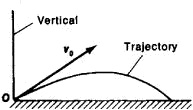Figure 1. A parabolic trajectory

Depending on the initial velocity, the trajectory of a particle moving in a central gravitational field may be an ellipse, a parabola, or a hyperbola; in special cases, the trajectory may be a straight line or a circle. If we regard the earth’s gravitational field as a central field and neglect atmospheric resistance, then the trajectory of a particle given a horizontally directed initial velocity v0 near the surface of the earth (Figure 2) is a circle when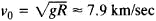(orbital velocity), an ellipse when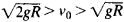, a parabola when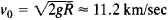(escape velocity), and a hyperbola when. Here, R is the earth’s radius, g is the acceleration of gravity near the earth’s surface, and the motion is considered with respect to axes that are undergoing translational motion, together with the center of the earth, relative to the stars.

In the case of a body, such as a satellite, all the above remarks apply to the trajectory of the body’s center of gravity. If, however, the direction of v0 is neither horizontal nor vertical and, the trajectory of the particle is an arc of an ellipse; this arc intersects the earth’s surface. The center of gravity of a ballistic missile has such a trajectory.

An example of a constrained particle is a small bob suspended on a string (seePENDULUM). If the string is deflected from the vertical and released without an initial velocity, the trajectory of the bob is an arc of a circle. If, however, an initial velocity that does not lie in the plane of the string’s deflection is imparted to the bob, the trajectories of the bob may be curves of rather complicated shape that lie on the surface of a sphere; such trajectories are characteristic of a spherical pendulum. In the special case of a conical pendulum, the trajectory of the bob is a circle lying in the horizontal plane.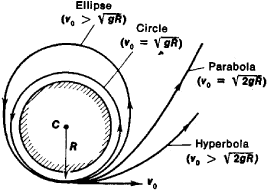Figure 2. Types of trajectories in the earth’s gravitational field

The trajectories of the points of a rigid body depend on the law of the body’s motion. For a translational motion of the body the trajectories of all its points are identical, but in all other cases the trajectories are generally different for the various points of the body. For example, for an automobile wheel on a rectilinear segment of a road, the trajectory of a point on the wheel’s rim relative to the highway is a cycloid, but the trajectory of the wheel’s center is a straight line. Relative to the automobile’s body, the trajectory of a point on the rim is a circle, but the wheel’s center is a fixed point.

The determination of trajectories is important both in theoretical studies and in the solution of many practical problems.

S. M. TARG

trajectory

[trə′jek·trē]
(geophysics)
The path followed by a seismic wave.
(mathematics)
A curve that intersects all the members of a given family of curves at the same angle.
(mechanics)
The curve described by an object moving through space, as of a meteor through the atmosphere, a planet around the sun, a projectile fired from a gun, or a rocket in flight.
McGraw-Hill Dictionary of Scientific & Technical Terms, 6E, Copyright © 2003 by The McGraw-Hill Companies, Inc.
References in periodicals archive ?
* This paper proposes a fast and efficient approach to get stay regions from daily trajectories based on a spatial and temporal context, then generate semantic trajectory by enriching these regions with semantic labels.
Managing a short-range trajectory requires a different skill set than, say, those we use to manipulate the stick and rudder.
In Section 2, we discuss the related works about trajectory data privacy protection.
Dajun, "Optimal trajectory analysis of hypersonic boost-glide waverider with heat load constraint", Aircraft Engineering and Aerospace Technology: An International Journal, vol.
Generated trajectory should allow the MR to going through the all base points in the specified sequence.
Most previous researchers typically focused on the mesh method for automated trajectory generation since such method is capable of generating trajectories on all types of complex workpieces [8, 11].
The trajectory filtering method based on the algorithm given in this article was validated.
Let [x.sub.*](*) [member of] [X.sub.p,r] be an arbitrary trajectory of the system (2.1) generated by the control function [u.sub.*](*) [member of] [U.sub.p,r].
The pulse-couple neural network is used in many application  and it is also applied into trajectory generation [12,13].
(1) Only one trajectory is included in the published trajectory dataset.
From the collected trajectories, a reference trajectory was generated for each exercise by using regression analysis as reported in .

Site: Follow: Share:
Open / Close